# Measuring Turbidity of Water to Determine Water Quality using Arduino and Turbidity Sensor

Published  October 5, 2020   1
M
Author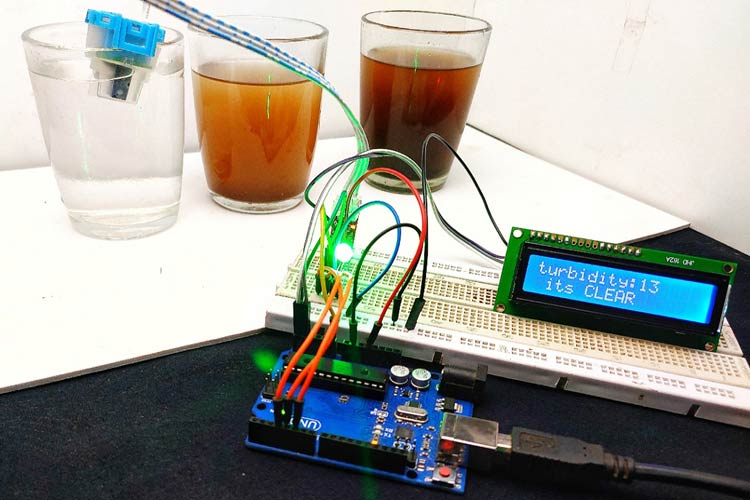When it comes to liquids, turbidity is an important term. Because it plays an important role in liquid dynamics and is also used to measure water quality. So in this tutorial, let's discuss what is turbidity, how to measure the turbidity of a liquid using Arduino. If you want to take this project further, you can also consider interfacing a pH meter with Arduino and also read the pH value of water to better assess the quality of water. Previously we have also built an IoT based Water quality monitoring device using ESP8266, you can also check that out if interested. That being said, let's get started

### What is Turbidity in Liquid?

Turbidity is the degree or level of cloudiness or haziness of a liquid. This happens due to the presence of large numbers of invisible particles (with the naked eye) similar to white smoke in the air. When light passes through liquids, light waves get scattered Due to the presence of these tiny particles. The turbidity of a liquid is directly proportional to the free suspended particles that is if the number of particles increases turbidity will also increase.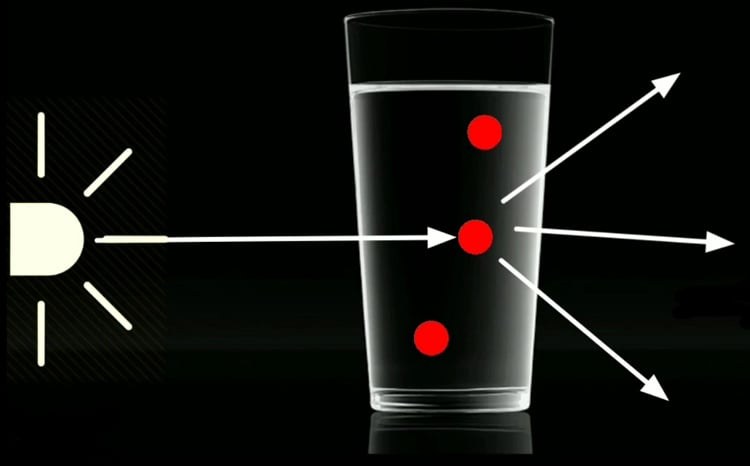### How to Measure Turbidity using Arduino?

As I mentioned earlier, turbidity happens due to the scattering of light waves, in order to measure the turbidity, we should measure the scattering of light. Turbidity is usually measured in nephelometric turbidity units (NTU) or Jackson turbidity units (JTLJ), depending on the method used for measurement. The two units are roughly equal.

Now let's see how a turbidity sensor works, it has two parts, transmitter and Receiver. The transmitter consists of a light source typically an led and a driver circuit. In the receiver end, there is a light detector like a photodiode or an LDR. We place the solution in between the transmitter and receiver.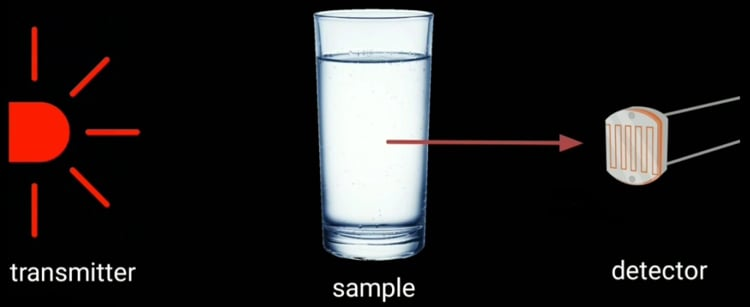Transmitter simply transmits the light, that light waves pass through the solution and the receiver receives the light. Normally (without the presence of a solution) the transmitted light completely receives on the receiver side. But in the presence of a turbid solution, the amount of transmitted light is very low. That is on the receiver side, we get only a low-intensity light and this intensity is inversely proportional to turbidity. So we can measure the turbidity by measuring the light intensity if the light intensity is high, the solution is less turbid and if the light intensity is very low that means the solution is more turbid.

### Components Needed for Making Turbidity Meter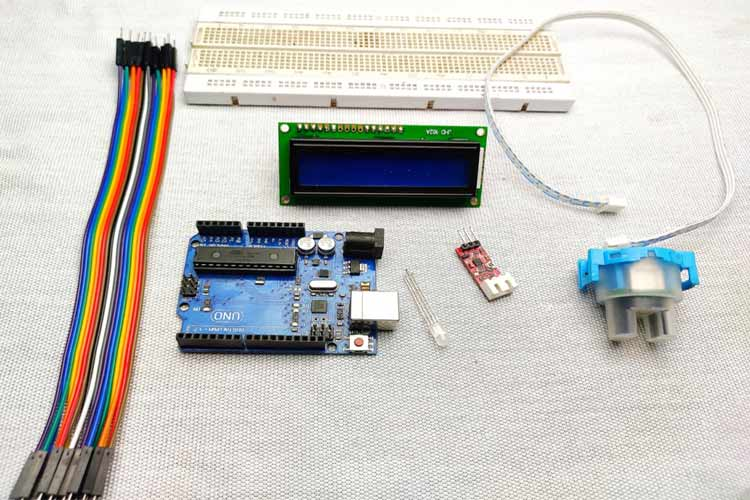1. Turbidity module
2. Arduino
3. 16*2 I2C LCD
4. Common cathode  RGB LED
6. Jumper wires

### Overview of Turbidity Sensor

The turbidity sensor used in this project is shown below.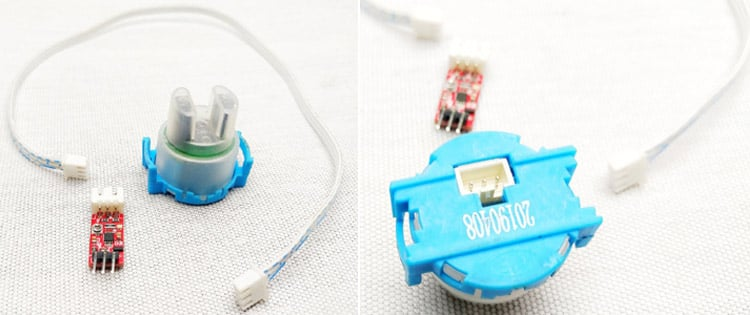As you can see, this turbidity sensor module comes with 3 parts. A waterproof lead, a driver circuit, and a connecting wire. The testing probe consists of both the transmitter and receiver.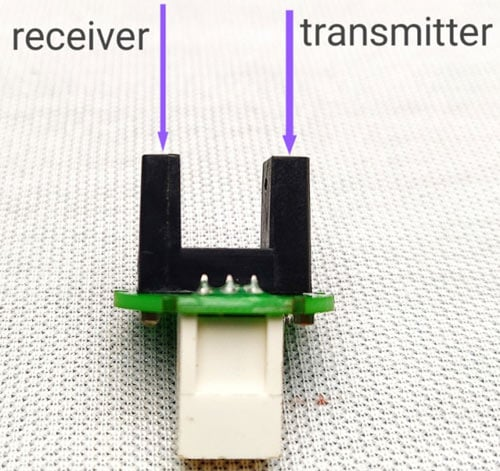The above image shows, this type of module uses an IR diode as a light source and an IR receiver as a detector. But the working principle is the same as before. The driver part (shown below) consists of an op-amp and some components which amplify the detected light signal.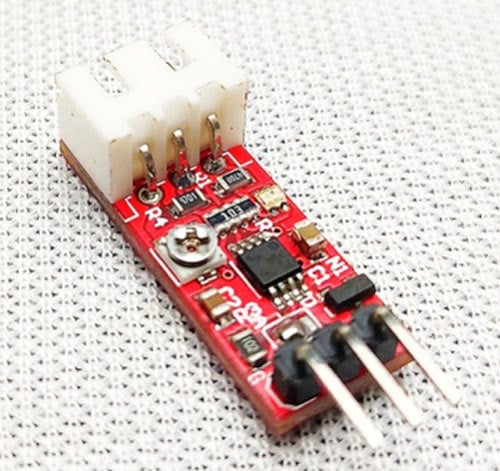The actual sensor can be connected to this module by using a JST XH connector. It has three pins, VCC, ground, and output. Vcc connects to the 5v and ground to ground. The output of this module is an analog value that is it changes according to the light intensity.

### Key Features of Turbidity Module

• Operating Voltage: 5VDC.
• Current: 30mA (MAX).
• Operating temperature: -30 ° C to 80 ° C.
• Compatible with Arduino, Raspberry Pi, AVR, PIC, etc.

### Interfacing Turbidity Sensor with Arduino – Circuit Diagram

The complete schematic to connect the Turbidity sensor to Arduino is shown below, the circuit was designed using EasyEDA.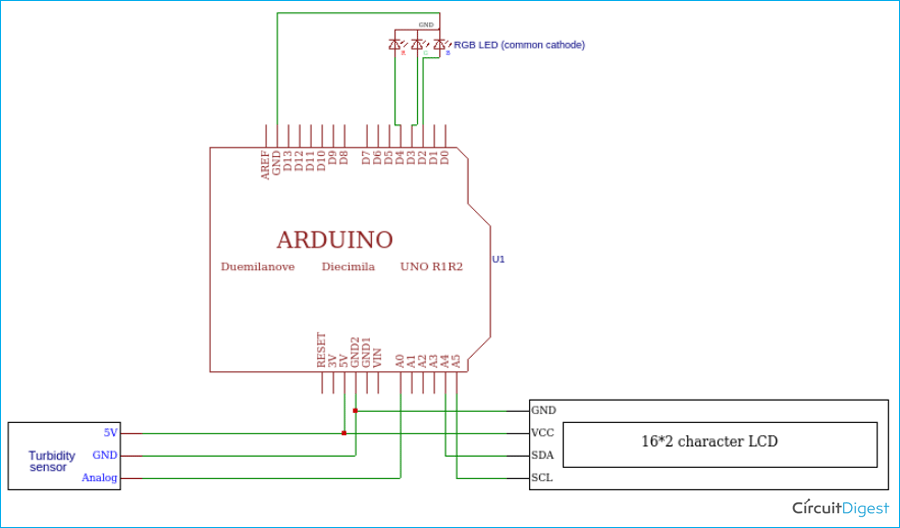This is a very simple circuit diagram. The output of the turbidity sensor is analog so that connected to Arduino's A0 pin, I2C LCD connected to I2C pins of Arduino that is SCL to A5 and SDA to A4. Then the RGB LED connected to digital pin D2, D3, and D4. After the connections are done, my hardware setup looks like this below.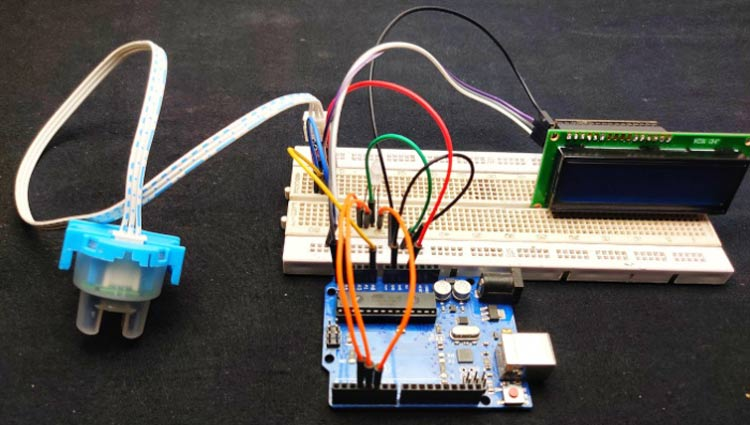Connect the VCC of the sensor to Arduino 5v, then connect ground to ground. The output pin of the sensor to analog 0 of Arduino. Next, connect VCC and ground of LCD module to 5v and ground of Arduino. Then SDA to A4 and SCL to A5, these two pins are the I2C pins of Arduino. finally connects the ground of RGB LED to the ground of Arduino and connect green to D3, blue to D4, and red to D5.

### Programming Arduino to Measure Turbidity in Water

The plan is to display the turbidity values from 0 to 100. That is the meter should display 0 for pure liquid and 100 for highly turbid ones. This Arduino code is also very simple and the complete code can be found at the bottom of this page.

First, I included the I2C liquid crystal library because we are using an I2C LCD to minimize the connections.

`#include <LiquidCrystal_I2C.h>`

Then I set integer for sensor input.

` int sensorPin = A0;`

In the setup section, I defined the pins.

```pinMode(3,OUTPUT);
pinMode(4,OUTPUT);
pinMode(5,OUTPUT);```

In the loop section, As I mentioned earlier, the output of the sensor is an analog value. So we need to read those values. With the help of the Arduino AnalogRead function, we can read the output values in the loop section.

`int sensorValue =analogRead(sensorPin);`

First, we need to understand the behavior of our sensor, which means we need to read the minimum value and maximum value of the turbidity sensor. we can read that value on the serial monitor using serial.println function.

To get these values, first, read the sensor freely that is without any solution. I got a value around 640 and after that, place a black substance between the transmitter and receiver, we get a value that is the minimum value, usually, that value is zero. So we got 640 as maximum and zero as a minimum. Now we need to convert these values to 0-100

For that, I used the map function of Arduino.

`int turbidity = map(sensorValue, 0,640, 100, 0);`

Then I displayed that values in the LCD display.

```  lcd.setCursor(0, 0);
lcd.print("turbidity:");
lcd.print("   ");
lcd.setCursor(10, 0);
lcd.print(turbidity); ```

After that, with the help of if conditions, I gave different conditions.

```if (turbidity < 20)
{
digitalWrite(2, HIGH);
digitalWrite(3, LOW);
digitalWrite(4, LOW);
lcd.setCursor(0, 1);
lcd.print(" its CLEAR ");
}```

This will active green led and display "its clear" on LCD if the turbidity value is below 20.

```if ((turbidity > 20) && (turbidity < 50))
{
digitalWrite(2, LOW);
digitalWrite(3, HIGH);
digitalWrite(4, LOW);
lcd.setCursor(0, 1);
lcd.print(" its CLOUDY ");
}```

This will active blue led and display "its cloudy" on LCD if the turbidity value is in between 20 and 50.

```if ((turbidity > 50)
{
digitalWrite(2, LOW);
digitalWrite(3, HIGH);
digitalWrite(4, LOW);
lcd.setCursor(0, 1);
lcd.print(" its DIRTY ");
}```

This will active red led and display "it's dirty" on LCD if the turbidity value is greater than 50 as shown below.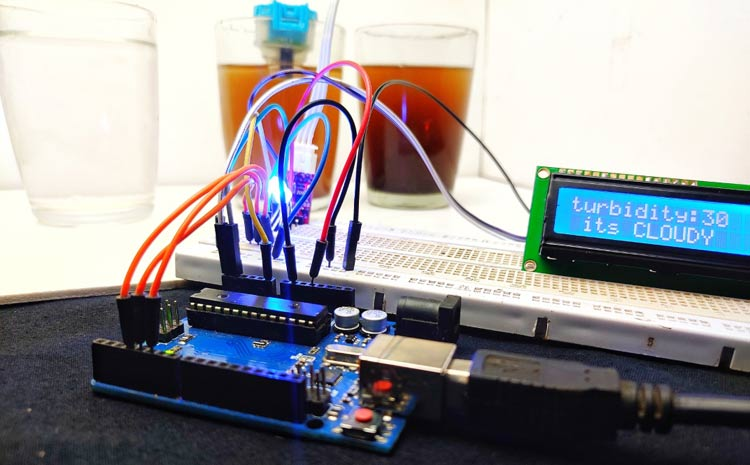Just follow the circuit diagram and upload the code, if everything goes correct, you should be able to measure the turbidity of water and the LCD should display the quality of the water as shown above.

Do note that this turbidity meter displays the percentage of turbidity and it might not be an accurate industrial value, but still it can be used to compare the water quality of two water. The complete working of this project can be found in the video below. Hope you enjoyed the tutorial and learn something useful if you have any question, you can leave them in the comment section below or use the CircuitDigest forums for posting your technical questions or start a relevant discussion.

Code
```#include <LiquidCrystal_I2C.h>
LiquidCrystal_I2C lcd(0x27, 2, 16);
int sensorPin = A0;
void setup()
{
Serial.begin(9600);
lcd.begin();
pinMode(2, OUTPUT);
pinMode(3, OUTPUT);
pinMode(4, OUTPUT);
}
void loop() {
Serial.println(sensorValue);
int turbidity = map(sensorValue, 0, 750, 100, 0);
delay(100);
lcd.setCursor(0, 0);
lcd.print("turbidity:");
lcd.print("   ");
lcd.setCursor(10, 0);
lcd.print(turbidity);
delay(100);
if (turbidity < 20) {
digitalWrite(2, HIGH);
digitalWrite(3, LOW);
digitalWrite(4, LOW);
lcd.setCursor(0, 1);
lcd.print(" its CLEAR ");
}
if ((turbidity > 20) && (turbidity < 50)) {
digitalWrite(2, LOW);
digitalWrite(3, HIGH);
digitalWrite(4, LOW);
lcd.setCursor(0, 1);
lcd.print(" its CLOUDY ");
}
if (turbidity > 50) {
digitalWrite(2, LOW);
digitalWrite(3, LOW);
digitalWrite(4, HIGH);
lcd.setCursor(0, 1);
lcd.print(" its DIRTY ");
}
}```
Video

Tags